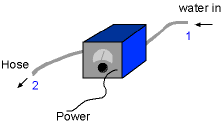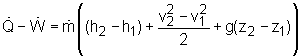Chapter 1. Basics 2. Pure Substances 3. First Law 4. Energy Analysis 5. Second Law 6. Entropy 7. Exergy Analysis 8. Gas Power Cyc 9. Brayton Cycle 10. Rankine Cycle Appendix Basic Math Units Thermo Tables Search eBooks Dynamics Fluids Math Mechanics Statics Thermodynamics Author(s): Meirong Huang Kurt Gramoll ©Kurt GramollTHERMODYNAMICS - CASE STUDY IntroductionJohn and his House John has a power washer, and plans to clean the siding of his house this weekend. John can not find the instruction of the power washer, hence he does not know the power needs for running it. What is known: Water enters the washer at 20 oC and 1 atm with a volumetric flow rate of 0.1 L/s through a 25-cm-diameter hose Water leaves the jet at 23 oC, 1 atm, and a velocity of 50 m/s at an elevation of 5 m The magnitude of the heat transfer rate from the power unit to the surroundings is 10% of the power input Question Determine the power input to the motor of the power washer. The power input is used to increase the enthalpy, kinetic energy, and potential energy of the water. Which one dominates power consumption? ApproachControl Volume Consider the power unit and the delivery hose as a control volume. "1" denotes the water at inlet and "2" denotes the water at exit The control volume is a one-inlet-one-exit system. So the energy balance equation is: